Biotechnology Model Test (2017)

# Biotechnology Model Test (2017) - IIT JAM

Test Description

## 60 Questions MCQ Test IIT JAM Past Year Papers and Model Test Paper (All Branches) - Biotechnology Model Test (2017)

Biotechnology Model Test (2017) for IIT JAM 2023 is part of IIT JAM Past Year Papers and Model Test Paper (All Branches) preparation. The Biotechnology Model Test (2017) questions and answers have been prepared according to the IIT JAM exam syllabus.The Biotechnology Model Test (2017) MCQs are made for IIT JAM 2023 Exam. Find important definitions, questions, notes, meanings, examples, exercises, MCQs and online tests for Biotechnology Model Test (2017) below.
Solutions of Biotechnology Model Test (2017) questions in English are available as part of our IIT JAM Past Year Papers and Model Test Paper (All Branches) for IIT JAM & Biotechnology Model Test (2017) solutions in Hindi for IIT JAM Past Year Papers and Model Test Paper (All Branches) course. Download more important topics, notes, lectures and mock test series for IIT JAM Exam by signing up for free. Attempt Biotechnology Model Test (2017) | 60 questions in 180 minutes | Mock test for IIT JAM preparation | Free important questions MCQ to study IIT JAM Past Year Papers and Model Test Paper (All Branches) for IIT JAM Exam | Download free PDF with solutions
 1 Crore+ students have signed up on EduRev. Have you?
Biotechnology Model Test (2017) - Question 1

### Which vitamins should be passed to the newborn for nutritional requirements ?

Biotechnology Model Test (2017) - Question 2

### Decreased growth, pigmented retinopathy, hemolytic anemia, and peripheral neuropathy are shown in 7- month- old boy. You surmise that these signs are the consequence of a vitamin deficiency leading to increased fatty acid oxidation. W hich of the following is most likely responsible for this patient's symptoms ?

Biotechnology Model Test (2017) - Question 3

### Cyclic photophosphorylation produces ________

Biotechnology Model Test (2017) - Question 4

Correct sequence of noncyclic photophosphorylation is ____

Biotechnology Model Test (2017) - Question 5

Common way that cell capture the energy liberated during the partitioning of large molecules is to add electrons to smaller, specialized molecules that can take them. This process of electron acceptance is otherwise recognized as ?

Biotechnology Model Test (2017) - Question 6

Choose the correct combination of components needed to fix up a polymerase chain reaction from the chase ?

Biotechnology Model Test (2017) - Question 7

In TCA cycle substrate level phosphorylation occurs at _______

Biotechnology Model Test (2017) - Question 8

How many ATP equivalents per mole of glucose input are required for gluconeogenesis ?

Biotechnology Model Test (2017) - Question 9

All of the following substances move across the plasma membrane of cells by passive diffusion EXCEPT

Biotechnology Model Test (2017) - Question 10

Loss of function mutations (involving a single allele) that involve the yield of an enzyme are usually autosomal ________ while loss of function mutations that affect structural proteins are usually autosomal ________

Biotechnology Model Test (2017) - Question 11

Order the following events in eukaryotic gene expression:

I. Binding of the 40S subunit

II. Creation of a 2'- 5' phosphodiester bond

III. Exporting RNA from the nucleus

IV. Binding of DNA Polymerase to the template strand

Biotechnology Model Test (2017) - Question 12

Which of the following proteins is NOT essential in the synthesis of eukaryotic proteins from DNA templates?

Biotechnology Model Test (2017) - Question 13

Mitochondrial DNA is inherited from?

Biotechnology Model Test (2017) - Question 14

The most accepted hypothesis of origin of life is _____

Biotechnology Model Test (2017) - Question 15

Bordeaux reagent is ______

Biotechnology Model Test (2017) - Question 16

pH of 0.001 M HCl is 3, on diluting 1ml of this to become 1 litre so what will be the pH ?

Biotechnology Model Test (2017) - Question 17

When phenol reacts with a mixture of conc. HNO3 and conc. H2SO4 the product formed is :

Biotechnology Model Test (2017) - Question 18

A compound x of formula C3H8O yield a compound C,H6O, on oxidation. To which of the following classes of compounds could x belong ?

Biotechnology Model Test (2017) - Question 19

The final product (c) formed in the reaction :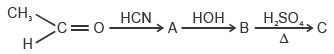Biotechnology Model Test (2017) - Question 20

Which one is not correct about perxenate ion (XeO64–) ?

Biotechnology Model Test (2017) - Question 21

A triangle with vertices (4, 0), (– 1, – 1), (3, 5) is

Biotechnology Model Test (2017) - Question 22

The domain of definition of the function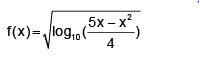Biotechnology Model Test (2017) - Question 23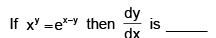Biotechnology Model Test (2017) - Question 24

A parallelepiped is formed by planes drawn through the points (2, 3, 5) and (5, 9, 7) parallel to the coordinate planes. The length of a diagonal of the parallelepiped is _____

Biotechnology Model Test (2017) - Question 25

If ω is an imaginary cube root of unity then (1 + ω – ω2)7 equals ________

Biotechnology Model Test (2017) - Question 26

A certain force applied to mass m1 given it an acceleration of 10 ms–2. The same force applied to mass m2 gives it an acceleration of 15ms–2. If the two masses are joined together and the same force is applied to the combination, the acceleration will be

Biotechnology Model Test (2017) - Question 27

An object will continue unaccelerating till _________

Biotechnology Model Test (2017) - Question 28

A train starts from station. A with uniform acceleration a for some distance and then goes with uniform retardation b for some more distance to come to rest at station B. The distance between station A and B is 4km and the train takes 4 minute to complete this journey. If a  and b  are in km (min)-2 then

Biotechnology Model Test (2017) - Question 29

A block of mass M is placed on an inclined plane of inclination 45°. The inclined plane moves horizontally with an acceleration of 10 ms–2 as shown in the fig. If acceleration due to gravity is 10 ms–2, the block will :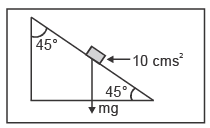Biotechnology Model Test (2017) - Question 30

If a person with a spring balance and a body hanging from it goes up and up in an aeroplane, then the reading of the weight of the body as indicated by the spring balance, will

*Multiple options can be correct
Biotechnology Model Test (2017) - Question 31

Correct statements regarding eukaryotic splicing are _______

*Multiple options can be correct
Biotechnology Model Test (2017) - Question 32

Which of the following is/are correct sources of Palmitic acid?

*Multiple options can be correct
Biotechnology Model Test (2017) - Question 33

Antifungal antibiotics are currently available for treating infectious disease. For example:

*Multiple options can be correct
Biotechnology Model Test (2017) - Question 34

The inactive form of the apoenzyme is known as a ______?

*Multiple options can be correct
Biotechnology Model Test (2017) - Question 35

Select the correct statements about the structure given below :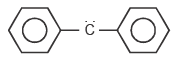*Multiple options can be correct
Biotechnology Model Test (2017) - Question 36

If mp, mn are masses of proton and neutron respectively and M1 and M2 are masses of 1210Ne and 4020Ca nucleus respectively, then ______

*Multiple options can be correct
Biotechnology Model Test (2017) - Question 37

If a hyperbola passes through the focus of the ellipse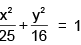and its transverse and conjugate axes coincide with the major minor axes of the ellipse, and the product of eccentricities is 1, then ________

*Multiple options can be correct
Biotechnology Model Test (2017) - Question 38

Internal bisector of ∠A of triangle ABC meets side BC at D. A line drawn through D perpendicular to AD intersects the side AC at E and the side AB at F. If a, b, c represent sides of DABC then

*Multiple options can be correct
Biotechnology Model Test (2017) - Question 39

In the given diagram, a line of force of a particular force field is shown. Out of the following options, it can never represent ________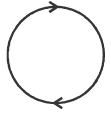*Multiple options can be correct
Biotechnology Model Test (2017) - Question 40

The electrostatic potential (φr) of a spherical symmetric system, kept at origin, is shown in the adjacent figure, and given as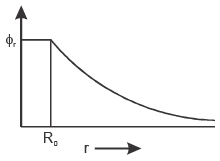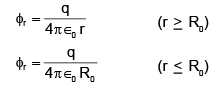W hich of the following option(s) is/are correct?

*Answer can only contain numeric values
Biotechnology Model Test (2017) - Question 41

The protein has a 3- fold symmetry axis, their rotation axis is _____

*Answer can only contain numeric values
Biotechnology Model Test (2017) - Question 42

Vm reveals the turnover number (kcat) of an enzyme if [Et] is known what is value of Kcat_____×105
sec–1.
if [Et] = 1 µM and Vm = 600 mols/L/sec, Kcat = ?

*Answer can only contain numeric values
Biotechnology Model Test (2017) - Question 43

In purple people eaters, one- horn is dominant and no horns is recessive, showing the cross of a purple people eater that is heterozygous for horns with a purple people eater that does not have horn. Summarize the genotypes & phenotype of the possible offspring ________ .

*Answer can only contain numeric values
Biotechnology Model Test (2017) - Question 44

In pea plants, spherical seeds (S) are dominant to dented seeds (s). In a genetic cross of two plants that are heterozygous for the seed shape trait, the offspring should have spherical seeds heterozygous (Ss) are________ .

*Answer can only contain numeric values
Biotechnology Model Test (2017) - Question 45

Genetic cross between two F1- hybrid pea plants having red seeds. Calculate the yield of pinkseeded plants in the F2 generation in______%.
Note: Red seeds are dominant to pink.

*Answer can only contain numeric values
Biotechnology Model Test (2017) - Question 46

At 300 K calculate the value of ΔG° _______ in kJ/mol.
Note that it is the same for both the substrate and the inhibitor at this temperature.

*Answer can only contain numeric values
Biotechnology Model Test (2017) - Question 47

Calculate the mass ratio of CH4 to O2 required for complete combustion________

*Answer can only contain numeric values
Biotechnology Model Test (2017) - Question 48

In E. coli DNA, the AT/GC ratio is 0.90. If the number of moles of A in the DNA sample is 560,000, calculate the number of moles of guanine present.

*Answer can only contain numeric values
Biotechnology Model Test (2017) - Question 49

pkeq. for the reaction :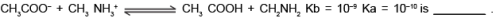*Answer can only contain numeric values
Biotechnology Model Test (2017) - Question 50

Number of electron pairs in the valence shell of central atom in I3- is _____

*Answer can only contain numeric values
Biotechnology Model Test (2017) - Question 51

Number of waves in an orbit of H- atom having radius equal to 8.464 × 10–10 m are ________.

*Answer can only contain numeric values
Biotechnology Model Test (2017) - Question 52

The total number of possible isomers for the complex compound [Cu(II)(NH3)4] [Pt(II)Cl4] is ________.

*Answer can only contain numeric values
Biotechnology Model Test (2017) - Question 53

In a class of 100 students there are 70 boys whose average marks in a subject are 75. If the average marks of the complete class is 72, then what is the average marks of the girls ?

*Answer can only contain numeric values
Biotechnology Model Test (2017) - Question 54

A and B play a game where each is asked to select a number from 1 to 25. If the two numbers match, both of them win a prize. The probability that they will not win a prize in a single trail is _________.

*Answer can only contain numeric values
Biotechnology Model Test (2017) - Question 55

Three lines 3x - 4y + 4 = 0 and 6x - 8y - 7 = 0 are tangent to the same circle. The radius of the circle is __________.

*Answer can only contain numeric values
Biotechnology Model Test (2017) - Question 56

Let Tn denote the number of triangles which can be formed using the vertices of a regular polygon of n sides. If Tn+1 – Tn = 21 then n equals _______.

*Answer can only contain numeric values
Biotechnology Model Test (2017) - Question 57

A particle moves from point (1, 0, 2.5) to the point (–2, 3, 4) m when a force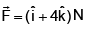acts on it. The work done on it is __________ J.

*Answer can only contain numeric values
Biotechnology Model Test (2017) - Question 58

A 500 kg car takes a round turn of radius 50 m with a velocity of 36 km/h. The centripetal force is ___________ N.

*Answer can only contain numeric values
Biotechnology Model Test (2017) - Question 59

The mass of hydrogen molecule is 3.32 × 10–27 Kg. If 1023 hydrogen molecules strike per second at 2 cm2 area of a rigid wall at an angle of 45° from the normal and rebound back with speed of 1000 m/s, then the pressure exerted on the wall is __________ Pascal.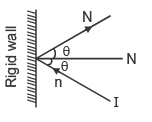*Answer can only contain numeric values
Biotechnology Model Test (2017) - Question 60

A wire has a resistance of 3.1W at 30°C and a resistance 4.5W  at 100°C. The temperature coefficient of the wire will be _____________ /°C

## IIT JAM Past Year Papers and Model Test Paper (All Branches)

8 docs|48 tests
Information about Biotechnology Model Test (2017) Page
In this test you can find the Exam questions for Biotechnology Model Test (2017) solved & explained in the simplest way possible. Besides giving Questions and answers for Biotechnology Model Test (2017), EduRev gives you an ample number of Online tests for practice

## IIT JAM Past Year Papers and Model Test Paper (All Branches)

8 docs|48 tests(Scan QR code)